### Knowing Our Numbers-Solutions Ex-1.2

CBSE Class –VI Mathematics
NCERT Solutions
Chapter 1 Knowing Our Numbers (Ex. 1.2)

Question 1. A book exhibition was held for four days in a school. The number of tickets sold at the counter on the first, second, third and final day was respectively 1094, 1812, 2050 and 2751. Find the total number of tickets sold on all the four days.
Answer: Number of tickets sold on first day = 1,094
Number of tickets sold on second day = 1,812
Number of tickets sold on third day = 2,050
Number of tickets sold on fourth day = 2,751
Total Number of tickets sold = 1094 + 1812 + 2050 + 2751 = 7,707
Therefore, 7,707 tickets were sold on all the four days.
Question 2. Shekhar is a famous cricket player. He has so far scored 6980 runs in test matches. He wishes to complete 10,000 runs. How many more runs does he need?
Answer: Runs to achieve = 10,000
Runs scored = 6,980
Runs required = 10,000 - 6980 = 3,020
Therefore, Shekar needs 3,020 more runs.
Question 3. In an election, the successful candidate registered 5,77,500 votes and his nearest rival secured 3,48,700 votes. By what margin did the successful candidate win the election?
Number of votes secured by his nearest rival =  3,48,700
Margin between them = 5,77,500 - 3,48,700 = 2,28,800
Therefore, the successful candidate won by a margin of 2,28,800 votes.
Question 4. Kirti Bookstore sold books worth 2,85,891 in the first week of June and books worth 4,00,768 in the second week of the month. How much was the sale for the two weeks together? In which week was the sale greater and by how much?
Answer: Books sold in first week = 2,85,891
Books sold in second week = 4,00,768
Total books sold = 2,85,891 + 4,00,768 = 6,86,659
Since, 4,00,768,> 2,85,891
Therefore sale of second week is greater than that of first week.
Books sold in second week = 4,00,768
Books sold in first week = 2,85,891
More books sold in second week =  4,00,768 - 2,85,891 = 1,14,877
Therefore, sale in second week was larger than sale in first week by 1,14,877
Question 5. Find the difference between the greatest and the least number that can be written using the digits 6, 2, 7, 4, 3 each only once.
Answer:  Greatest five-digit number using digits 6,2,7,4,3 = 76432
Smallest five-digit number using digits 6,2,7,4,3 = 23467
Difference = 76432 - 23467= 52965
Therefore the difference is 52965.
Question 6. A machine, on an average, manufactures 2,825 screws a day. How many screws did it produce in the month of January 2006?
Answer: Number of screws manufactured in one day = 2,825
Number of days in the month of January (31 days) = 2,825 x 31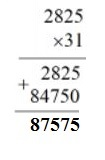Therefore the machine produced 87,575 screws in the month of January.
Question 7. A merchant had 78,592 with her. She placed an order for purchasing 40 radio sets at 1,200 each. How much money will remain with her after the purchase?
Cost of 40 radios = 1200 x 40 = 48,000
Now, Total money with merchant = 78,592
Money spent by her = 48,000
Money left with her = 78,592 - 40,000 = 30,592
Therefore, Rs 30,592 will remain with her after the purchase.
Question 8. A student multiplied 7236 by 65 instead of multiplying by 56. By how much was his answer greater than the correct answer?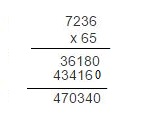Correct answer = 7236 x 56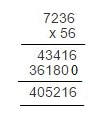Difference in answers = 470340 – 405216 = 65,124
Question 9. To stitch a shirt 2 m 15 cm cloth is needed. Out of 40 m cloth, how many shirts can be stitched and how much cloth will remain?
Answer: Cloth required to stitch one shirt = 2 m 15 cm
= 2 x 100 cm + 15 cm = 215 cm
Length of cloth = 40 m = 40 x 100 cm = 4000 cm
Number of shirts can be stitched = 4000 $÷$ 215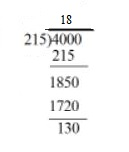Therefore, 18 shirts can be stitched and 130 cm (1 m 30 cm) cloth will remain.
Question 10. Medicine is packed in boxes, each weighing 4 kg 500 g. How many such boxes can be loaded in a can which cannot carry beyond 800 kg?
Answer: The weight of one box = 4 kg 500 g = 4 x 1000 g + 500 g = 4500 g
Maximum load can be loaded in van = 800 kg = 800 x 1000 g = 800000 g
Number of boxes = 800000 $÷$ 4500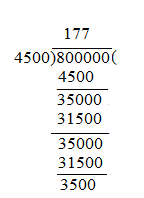Therefore, 177 boxes can be loaded.
Question 11. The distance between the school and the house of a student’s house is 1 km 875 m. Everyday she walks both ways. Find the total distance covered by her in six days.
Answer: Distance between school and home = 1.875 km
Distance between home and school = + 1.875 km
Total distance covered in one day = 3.750 km
Distance covered in six days = 3.750 x 6 = 22.500 km
Therefore, 22 km 500 m distance covered in six days.
Question 12. A vessel has 4 liters and 500 ml of curd. In how many glasses each of 25 ml capacity, can it be filled?
Answer: Capacity of curd in a vessel = 4 liters 500 ml = 4 x 1000 ml + 500 ml = 4500 ml
Capacity of one glass = 25 ml
Number of glasses can be filled = 4500 $÷$ 25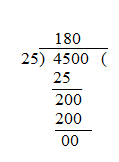Therefore, 180 glasses can be filled by curd.